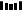### 题目描述

输入: piles = [3,6,7,11], H = 8



输入: piles = [30,11,23,4,20], H = 5



• 1 <= piles.length <= 10^4
• piles.length <= H <= 10^9
• 1 <= piles[i] <= 10^9

### 代码实现

class Solution {
public int minEatingSpeed(int[] piles, int H) {
int low = 1;
int high = pow(10, 9);
int mid;
while (low < high) {
mid = (low + high) / 2;
if (!canPlayGame(piles, mid, H)) {
low = mid + 1;
}
else {
high = mid;
}
}
return low;

}
private boolean canPlayGame(int[] piles, int k, int H) {
int t = 0;
for (int i: piles) {
if (i % k != 0) {
t++;
}
t += i / k;
}
return t <= H;
}
}1.程序员

2.【GitHub

3.【算法动画：七分钟理解什么是KMP算法

4.【数据结构十大经典排序算法动画与解析，看我就够了！▼ 点击『阅读原文』解锁更多图解 LeetCode 题目